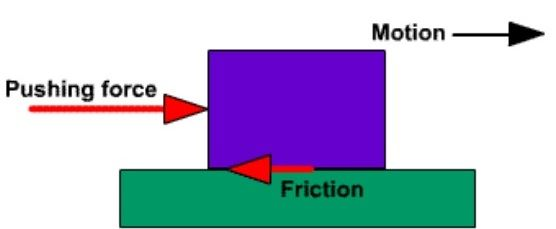# Kinetic Friction

## What is Kinetic Friction?

Kinetic friction is defined as a force that acts between moving surfaces. A body moving on the surface experiences a force in the opposite direction of its movement. The magnitude of the force will depend on the coefficient of kinetic friction between the two material.

Friction is easily defined as the force that holds back a sliding object. The kinetic friction is a part of everything and it interferes motion of two or more objects. The force acts in the opposite direction to the way an object wants to slide. If a car has to stop, we apply brakes and that is exactly where the friction comes into play. While walking, when one wants to suddenly come to a halt, friction is to thank again. But when we have to stop in the middle of a puddle, things get harder since friction is less there and cannot aid one so much.A Model For Kinetic Friction

## Kinetic Friction Formula

The coefficient of kinetic friction is denoted by the Greek letter “mu” (μ), with a subscript “k”. The force of kinetic friction is μk times the normal force on a body. It is expressed in Newtons (N). The kinetic friction equation can be written as:

Force of kinetic friction = (coefficient of kinetic friction)(normal force)

 Fk= μk η
Where,
• Fk = force of kinetic friction
• μk = coefficient of kinetic friction
• η = normal force (Greek letter “eta”)

### Types of Friction

There are two main types of friction which are mentioned in the points below.

• Static Friction
• Kinetic Friction.

Static friction is the one present between two objects that are not moving with respect to each other. In liquids, friction is the resistance between moving layers of a fluid better known as viscosity. Now take two moving solid objects, this is known as moving or kinetic friction due to its motion.

This friction is a particular fraction of the perpendicular force acting between two bodies. A coefficient of friction determines the fraction which in turns determined through experiments. The force is independent of the contact area and doesn’t change no matter how fast the two objects are moving. While in contrast, static friction is what affects stationary objects. The force of friction is a force that resists motion when two objects are in contact. If you look at the surfaces of all objects, there are tiny bumps and ridges. Those microscopic peaks and valleys catch on one another when two objects are moving past each other. There are of course other forces at work like chemical bonding and electrical interactions.

## Applications of Kinetic Friction

• Friction also plays a huge role in everyday occurrences like while rubbing of two objects takes place. The resulting motion converts into heat and thus resulting in the fire in some instances.
• It is also responsible for wear and tear and that’s why we need oil to lubricate machine parts, as it reduces friction.
• When two objects are rubbed against each other, the frictional force is converted into thermal energy, in few cases giving rise to fire
• Kinetic friction is responsible for the wear and tear of machine parts, hence it is important to lubricate the machine parts with oil.### What is the Difference Between Static Friction and Kinetic Friction?

 Static Friction Kinetic Friction Static friction is the friction present between two or more objects that are not moving with respect to each other Kinetic friction is the friction present between two or more objects that are in motion with respect to each other. The magnitude of static friction is greater due to the greater value of its coefficient The magnitude of the kinetic friction is comparatively lesser due to the low value of its coefficient The equation representing static friction is given by $$\begin{array}{l}F_{s}=\mu_{s}\eta\end{array}$$ The equation representing kinetic friction is given by $$\begin{array}{l}F_{k}=\mu_{k}\eta\end{array}$$

The video explains the role of friction in walking with the help of animations.## Frequently Asked Questions – FAQs

### What is friction?

Friction is the force that opposes the rolling or sliding of one solid body over another.

### What are the two types of friction?

Static friction and kinetic friction.

### What is meant by kinetic friction?

Kinetic friction is the friction that acts between moving surfaces.

### What is meant by static friction?

Static friction is the friction that acts between two stationary surfaces.

Stay tuned with BYJU’S to learn more physics concepts with the help of interactive video lessons.

Test your knowledge on Kinetic friction